# Single Correct MCQ Of Functions, Past Year Questions JEE Advance, Class 12, Maths

## 33 Questions MCQ Test Maths 35 Years JEE Mains & Advance Past year Papers Class 12 | Single Correct MCQ Of Functions, Past Year Questions JEE Advance, Class 12, Maths

Description
This mock test of Single Correct MCQ Of Functions, Past Year Questions JEE Advance, Class 12, Maths for JEE helps you for every JEE entrance exam. This contains 33 Multiple Choice Questions for JEE Single Correct MCQ Of Functions, Past Year Questions JEE Advance, Class 12, Maths (mcq) to study with solutions a complete question bank. The solved questions answers in this Single Correct MCQ Of Functions, Past Year Questions JEE Advance, Class 12, Maths quiz give you a good mix of easy questions and tough questions. JEE students definitely take this Single Correct MCQ Of Functions, Past Year Questions JEE Advance, Class 12, Maths exercise for a better result in the exam. You can find other Single Correct MCQ Of Functions, Past Year Questions JEE Advance, Class 12, Maths extra questions, long questions & short questions for JEE on EduRev as well by searching above.
QUESTION: 1

### Let R be the set of real numbers. If f : R → R is a function defined by f (x) = x2, then f is :

Solution:

f (x) = x2 is many one as f (1) = f (–1) = 1 Also f is into as – ve real number have no pre-image.
∴ F is neither injective nor surjective.

QUESTION: 2

### The entire graphs of the equation y = x2 + kx – x + 9 is strictly above the x-axis if and only if

Solution: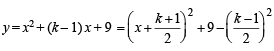For entire graph to be above x-axis, we should have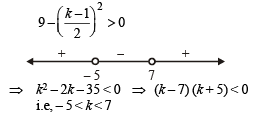QUESTION: 3

### Let f(x) = | x – 1 |. Then

Solution: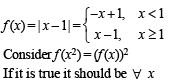∴ Put x = 2
LHS = f (22) = |4 – 1| = 3 and RHS = ( f (2))2 = 1
∴ (a) is not correct
Consider f (x + y) = f (x) + f (y)
Put x = 2, y = 5 we get
f (7) = 6; f (2) + f (5) = 1 + 4 = 5
∴ (b) is not correct
Consider f (| x |) = | f (x) |
Put x = – 5 then f (| –5 |) = f (5) = 4
| f (– 5) | = | – 5 – 1| = 6
∴ (c) is not correct.
Hence (d) is the correct alternative.

QUESTION: 4

If x satisfies |x - 1| + |x - 2| + |x - 3| > 6 , then

Solution: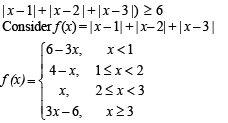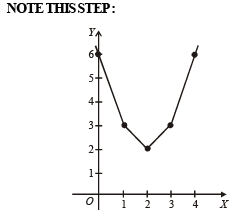Graph of f (x) shows f (x) > 6 for x < 0 or x > 4

QUESTION: 5

If f ( x) = cos(ln x), then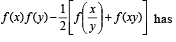the value

Solution: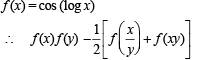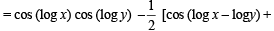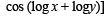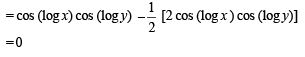QUESTION: 6

The domain of definition of the function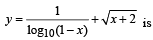Solution: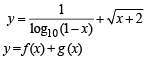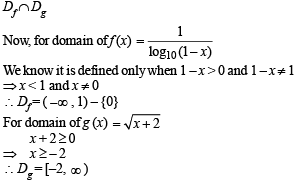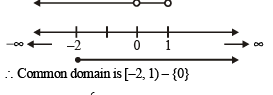QUESTION: 7

Which of the following functions is periodic?

Solution: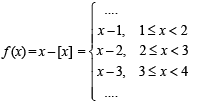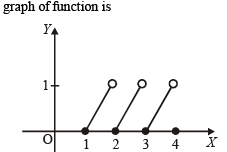Clearly it is a periodic function with period 1.
∴ (a) is the correct alternative.

QUESTION: 8

Let f(x) = sin x and g(x) = ln | x |. If th e ran ges of th e composition functions fog and gof are R1 and Rrespectively, th en

Solution: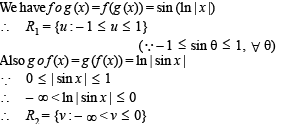QUESTION: 9

Let f ( x) = ( x + 1)2 - 1,x > -1 . Th en th e set {x : f (x) = f -1 (x)} is

Solution: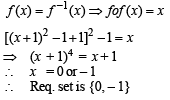QUESTION: 10

Th e fun ction f(x) = |px – q| + r | x |, x ∈ (-∞,∞) where p > 0, q > 0, r > 0  assumes its minimum value only on one point if

Solution: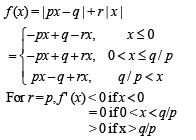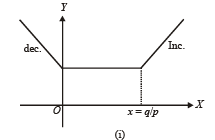From graph (i) infinite many points for min value of f (x)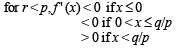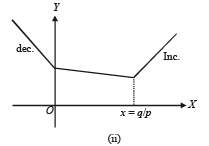From graph (ii) only pt. of min of f (x) at x = q/p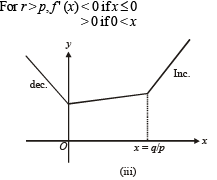From graph (iii) only one pt. of min of f (x) at x = 0

QUESTION: 11

Let f(x) be defined for all x > 0 and be continuous. Let f(x)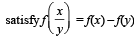for all x, y and f(e) = 1. Then

Solution:

f (x) is continuous and defined for all x > 0 and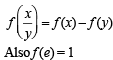⇒ Clearly f (x) = ln x which satisfies all these properties
∴ f (x) = ℓnx

QUESTION: 12

If the function f: [1, ∞) → [1, ∞) is defined by f(x) = 2x (x-1), then f–1 (x) is

Solution: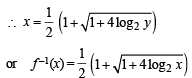QUESTION: 13

Let f : R → R be any function. Define g : R → R by g(x) = |f(x)| for all x.  Then g is

Solution:

Let h (x) = |x| then g (x) = |f (x)| = h (f (x))
Since composition of two continuous functions is continuous, therefore g is continuous if f is continuous.

QUESTION: 14

The domain of definition of the function f(x) given by the equation 2x + 2y = 2 is

Solution: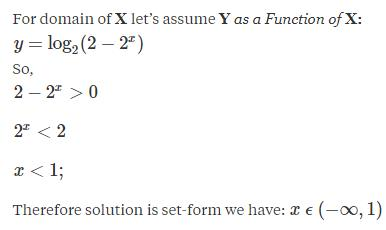QUESTION: 15

Let g(x) = 1 + x - [x] and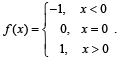Then for allx, f(g(x)) is equal to

Solution: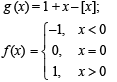For integral values of x; g (x) = 1
For x < 0; (but not integral value) x – [x] > 0 ⇒ g (x) > 1
For x > 0;(but not integral value) x – [x] > 0 ⇒ g (x) >1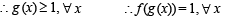QUESTION: 16

If f:[1, ∞) → [2, ∞) is given by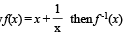equals

Solution: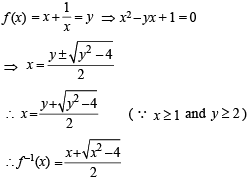QUESTION: 17

The domain of definition of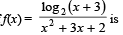Solution: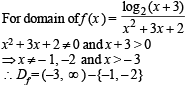QUESTION: 18

Let E = {1, 2, 3, 4} and F = {1, 2}. Then the number of onto functions from E to F is

Solution:

From E to F we can define, in all, 2 × 2 × 2 × 2 = 16 functions (2 options for each element of E) out of which 2 are into, when all the elements  of E either map to 1 or to 2.
∴ No. of onto functions = 16  – 2 = 14

QUESTION: 19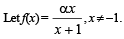Then, for what value of a is f (f(x)) = x ?

Solution: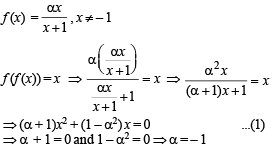QUESTION: 20

Suppose f(x) = (x + 1)2 for x > -1. If g(x) is the function whose graph is the reflection of the graph of f (x) with respect to the line y = x, then g(x) equals

Solution:

Given that f (x) = (x + 1)2, x > – 1
Now if g (x) is the reflection of f (x) in the line y = x then it can be obtained by interchanging x and y in f (x) i.e., y = (x + 1)2 changes to x = (y + 1)2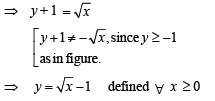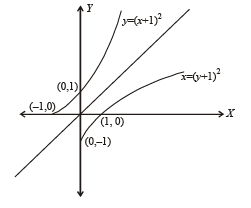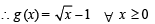QUESTION: 21

Let f unction f : R → R be defined by f(x) = 2x + sin x for x ∈ R , then f is

Solution: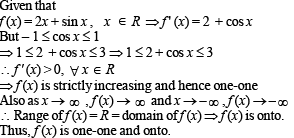QUESTION: 22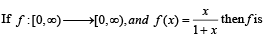Solution: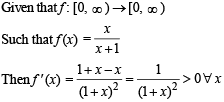∴ f is an increasing function ⇒ f is one-one.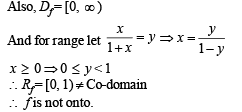QUESTION: 23

Domain of definition of the function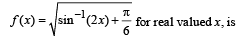Solution: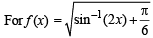to be defined and real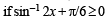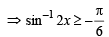...(1) But we know that – π/2 < sin–1 2x < π/2 ...(2) Combining (1) and (2), we get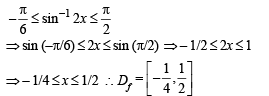QUESTION: 24

Range of the function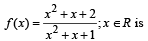Solution:

We have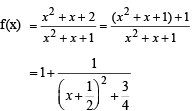We can see here that as x → ∞ , f (x) → 1 which is the min value of f (x). i.e. fmin = 1. Also f (x) is max when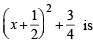min which is so when x  = – 1/2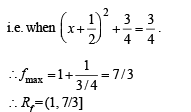QUESTION: 25

If f (x) = x2 + 2bx + 2c2 and g (x) = - x2 -2cx+ b2 such that min f (x) > max g (x), then the relation between b and c, is

Solution: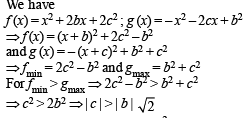QUESTION: 26

If f(x) = sin x + cos x, g (x) = x2 – 1, then g (f(x)) is invertible in the domain

Solution: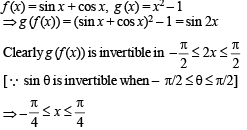QUESTION: 27

If the functions f(x) and g(x) are defined on R → R such that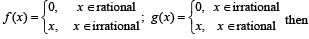Solution: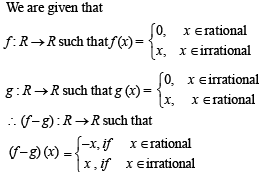Since f  –  g : R → R for any x there is only one value of (f (x) – g(x)) whether x is rational or irrational.
Moreover as x∈R, f (x) – g (x) also belongs to R.
Therefore, (f – g) is one-one onto.

QUESTION: 28

X an d Y are two sets and f : X → Y. If {f(c) = y; c ⊂ X, y ⊂ Y} and {f–1(d) = x; d ⊂ Y, x ⊂ X}, then the true statement is

Solution:

Given that X and Y are two sets and f : X → Y.
{f (c) = y; c ⊂ X, y ⊂ Y} and
{f –1(d) = x : d ⊂ Y, x ⊂ X }
The pictorial representation of given information is as shown: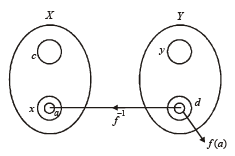Since f –1 (d) =  x ⇒ f (x) = d Now if a ⊂ x
⇒ f (a) ⊂ f (x) = d ⇒ f –1 [f (a)]  = a
∴ f –1 (f (a)) = a, a ⊂ x is the correct option.

QUESTION: 29

If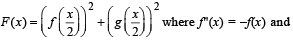g(x) = f '(x) and given that F(5) = 5, then F(10) is equal to

Solution: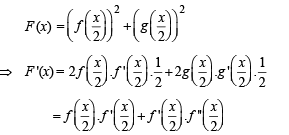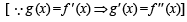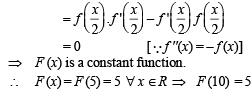QUESTION: 30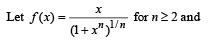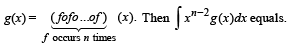Solution: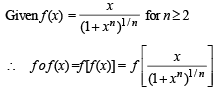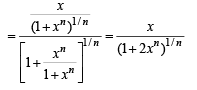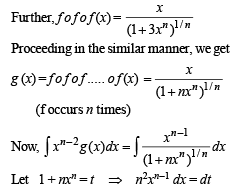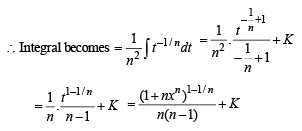QUESTION: 31

Let f, g and h be real-valued functions defined on the interval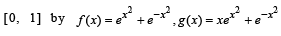and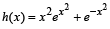. If a, b and c denote, respectively, the absolute maximum of f, g and h on [0, 1], then

Solution: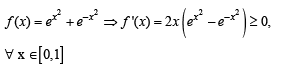∴ f (x) is an increasing function on [ 0,1]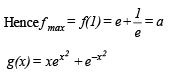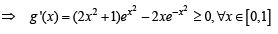∴ g (x) is an increasing function on [ 0,1]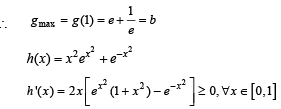∴ h (x) is an increasing function on [ 0,1]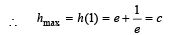Hence a = b = c.

QUESTION: 32

Let f (x) = x2 and g(x) = sin x for all x ∈ R. Then the set of all x satisfying (f o g o g o f) (x) = (g o g o f) (x), where (f o g) (x) = f (g(x)), is

Solution: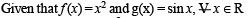Then (gof) (x) = sin x2
⇒ (gogof) (x) = sin (sin x2)
⇒ (fogogof) (x) = sin 2 (sin x2) As given that (fogogof) (x) = (gogof) (x)
⇒ sin2 (sin x2) = sin (sin x2)
⇒ sin (sin x2) = 0,1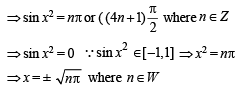QUESTION: 33

Th e function f : [0, 3] → [1, 29], defi n ed by f(x) = 2x3  – 15x2 + 36x + 1, is

Solution:

We have  f(x) = 2x3 – 15x2 + 36x + 1
⇒ f (x') = 6x2 – 30x + 36
=  6(x2 – 5x + 6)
=  6 (x – 2) (x – 3)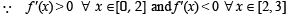∴ f(x) is in creasing on [0, 2] and decreasing on [2, 3]
∴ f(x) is many one on [0, 3]
Also f(0) = 1, f(2) = 29, f(3) = 28
∴ Global min = 1 and Global max = 29 i.e.,  Range of f = [1, 29] = codomain
∴ f is onto.